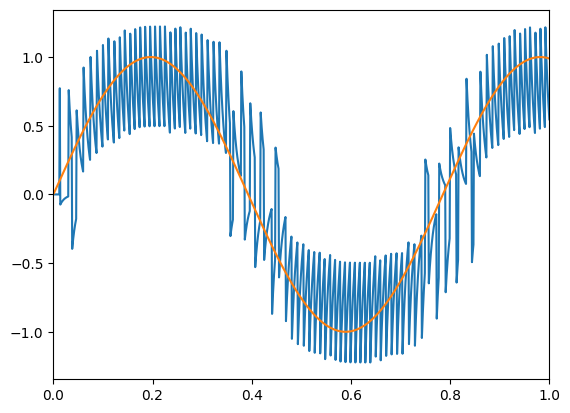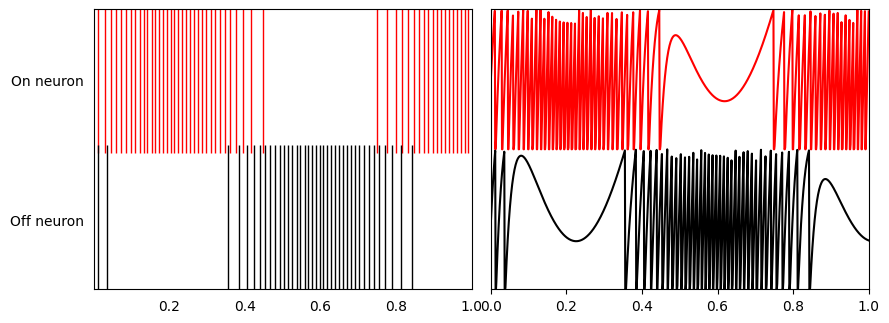Note

This documentation is for a development version. Click here for the latest stable release (v4.0.0).

# Two neurons¶

This demo shows how to construct and manipulate a complementary pair of neurons.

These are leaky integrate-and-fire (LIF) neurons. The neuron tuning properties have been selected so there is one ‘on’ and one ‘off’ neuron.

One neuron will increase for positive input, and the other will decrease. This can be thought of as the simplest population that is able to give a reasonable representation of a scalar value.

:

%matplotlib inline
import matplotlib.pyplot as plt
import numpy as np

import nengo
from nengo.dists import Uniform
from nengo.utils.matplotlib import rasterplot


## Step 1: Create the neurons¶

:

model = nengo.Network(label="Two Neurons")
with model:
neurons = nengo.Ensemble(
2,
dimensions=1,  # Representing a scalar
intercepts=Uniform(-0.5, -0.5),  # Set the intercepts at .5
max_rates=Uniform(100, 100),  # Set the max firing rate at 100hz
encoders=[, [-1]],
)  # One 'on' and one 'off' neuron


## Step 2: Create input for the model¶

Create an input node generating a sine wave.

:

with model:
sin = nengo.Node(lambda t: np.sin(8 * t))


## Step 3: Connect the network elements¶

:

with model:
nengo.Connection(sin, neurons, synapse=0.01)


## Step 4: Probe outputs¶

Anything that is probed will collect the data it produces over time, allowing us to analyze and visualize it later.

:

with model:
sin_probe = nengo.Probe(sin)  # The original input
spikes = nengo.Probe(neurons.neurons)  # Raw spikes from each neuron
# Subthreshold soma voltages of the neurons
voltage = nengo.Probe(neurons.neurons, "voltage")
# Spikes filtered by a 10ms post-synaptic filter
filtered = nengo.Probe(neurons, synapse=0.01)


## Step 5: Run the model¶

:

with nengo.Simulator(model) as sim:  # Create a simulator
sim.run(1)  # Run it for 1 second


## Step 6: Plot the results¶

:

t = sim.trange()

# Plot the decoded output of the ensemble
plt.figure()
plt.plot(t, sim.data[filtered])
plt.plot(t, sim.data[sin_probe])
plt.xlim(0, 1)

# Plot the spiking output of the ensemble
plt.figure(figsize=(10, 8))
plt.subplot(2, 2, 1)
rasterplot(t, sim.data[spikes], colors=[(1, 0, 0), (0, 0, 0)])
plt.yticks((1, 2), ("On neuron", "Off neuron"))
plt.ylim(2.5, 0.5)

# Plot the soma voltages of the neurons
plt.subplot(2, 2, 2)
plt.plot(t, sim.data[voltage][:, 0] + 1, "r")
plt.plot(t, sim.data[voltage][:, 1], "k")
plt.yticks(())
plt.axis([0, 1, 0, 2])